## Nonlinear Dynamic Systems

### Nonlinear functions

#### What's a linear function?

Well, gee Mikey, it's one that can be written in the form of a straight line. Remember the formula ...
y = mx + b
where m is the slope and b is the y-intercept?

#### What's a nonlinear function?

Any function that ain't linear!
Is the Alpha model a linear model?

Yes, because qn+1 is a linear function of qn

But wait! Its output, the plot of its behavior over time (its time series shown earlier) is not a straight line. Doesn't that make it a nonlinear system?
No, what makes a dynamic system nonlinear ....
is whether the function specifying the change is nonlinear. Not whether its behavior is nonlinear.
And y is a nonlinear function of x if x is multiplied by another (non-constant) variable, or multiplied by itself (i. e., raised to some power).
We illustrate nonlinear systems using ...

### Logistic Difference Equation

... a model often used to introduce chaos. The Logistic Difference Equation, or Logistic Map, though simple, displays the major chaotic concepts.

#### Growth model

We start, generally, with a model of growth.

xnew = r xold

We prefer to write this in terms of n:

xn+1 = r xn.

This says x changes from one time period, n, to the next, n+1, according to r. If r is larger than one, x gets larger with successive iterations If r is less than one, x diminishes. (In the "Alice" example at the beginning, r is .5).
Let's set r to be larger than one...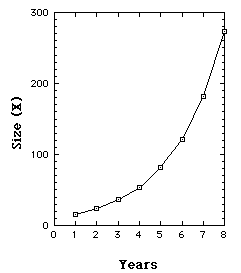We start, year 1 (n=1), with a population of 16 [x1=16], and since r=1.5, each year x is increased by 50%. So years 2, 3, 4, 5, ... have magnitudes 24, 36, 54, ...
Our population is growing exponentially. By year 25 we have over a quarter million.

Iterations of Growth model with r = 1.5

So far, notice, we have a linear model that produces unlimited growth.

#### Limited Growth model - Logistic Map.

The Logistic Map prevents unlimited growth by inhibiting growth whenever it achieves a high level. This is achieved with an additional term, [1 - xn].
The growth measure (x) is also rescaled so that the maximum value x can achieve is transformed to 1. (So if the maximum size is 25 million, say, x is expressed as a proportion of that maximum.)
Our new model is

xn+1 = r xn [1 - xn]

[r between 0 and 4.]
The [1-xn] term serves to inhibit growth because as x approaches 1, [1-xn] approaches 0.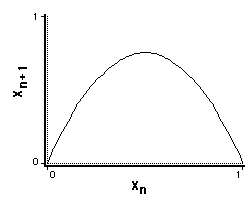Plotting xn+1 vs. xn, we see we have a nonlinear relation.
Limited growth (Verhulst) model. Xn+1 vs. xn, r = 3.

We have to iterate this function to see how it will behave ...
Suppose r=3, and x1=.1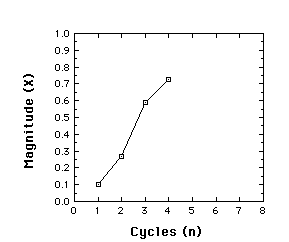x2 = rx1[1-x1] = 3(.1)(.9) = .27

x3= r x2[1-x2]= 3(.27)(.73) = .591

x4= r x3[1-3]= 3(.591)(.409) = .725

Behavior of the Logistic map for r = 3, x1 = .1, iterated to give x2, x3, and x4
It turns out that the logistic map is a very different animal, depending on its control parameter r. To see this, we next examine the time series produced at different values of r, starting near 0 and ending at r=4. Along the way we see very different results, revealing and introducing major features of a chaotic system.

#### When r is less than 1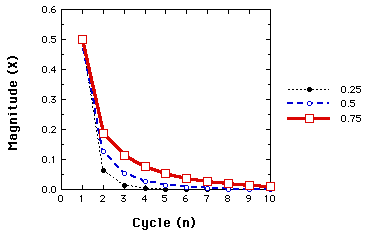Behavior of the Logistic map for r=.25, .50, and .75. In all cases x1=.5.

The same fates awaits any starting value. So long as r is less than 1, x goes toward 0. This illustrates a one-point attractor.

#### When r is between 1 and 3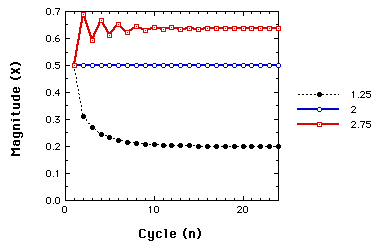Behavior of the Logistic map for r=1.25, 2.00, and 2.75. In all cases x1=.5.

Now, regardless, of the starting value, we have non-zero one-point attractors.

#### When r is larger than 3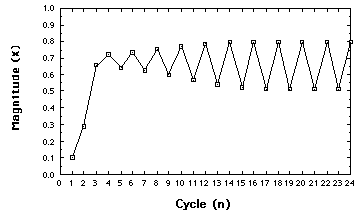Behavior of the Logistic map for r=3.2.

Moving just beyond r=3, the system settles down to alternating between two points. We have a two-point attractor. We have illustrated a bifurcation, or period doubling,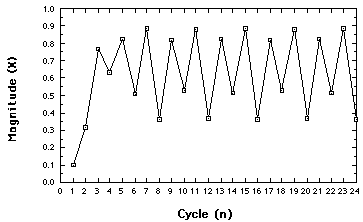Behavior of the Logistic map for r= 3.54. Four-point attractor

Another bifurcation. The concept: an N-point attractor.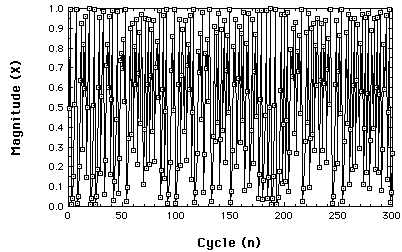Chaotic behavior of the Logistic map at r= 3.99.

So, what is an attractor? Whatever the system "settles down to".
Here is a very important concept from nonlinear dynamics: A system eventually "settles down". But what it settles down to, its attractor, need not have 'stability'; it can be very 'strange'.